Main Content

# histogram

Histogram plot

## Description

Histograms are a type of bar plot for numeric data that group the data into bins. After you create a `Histogram` object, you can modify aspects of the histogram by changing its property values. This is particularly useful for quickly modifying the properties of the bins or changing the display.

## Creation

### Syntax

``histogram(X)``
``histogram(X,nbins)``
``histogram(X,edges)``
``histogram('BinEdges',edges,'BinCounts',counts)``
``histogram(C)``
``histogram(C,Categories)``
``histogram('Categories',Categories,'BinCounts',counts)``
``histogram(___,Name,Value)``
``histogram(ax,___)``
``h = histogram(___)``

### Description

example

````histogram(X)` creates a histogram plot of `X`. The `histogram` function uses an automatic binning algorithm that returns bins with a uniform width, chosen to cover the range of elements in `X` and reveal the underlying shape of the distribution. `histogram` displays the bins as rectangles such that the height of each rectangle indicates the number of elements in the bin.```

example

````histogram(X,nbins)` uses a number of bins specified by the scalar, `nbins`.```

example

````histogram(X,edges)` sorts `X` into bins with the bin edges specified by the vector, `edges`. Each bin includes the left edge, but does not include the right edge, except for the last bin which includes both edges.```
````histogram('BinEdges',edges,'BinCounts',counts)` manually specifies bin edges and associated bin counts. `histogram` plots the specified bin counts and does not do any data binning.```

example

````histogram(C)`, where `C` is a categorical array, plots a histogram with a bar for each category in `C`.```
````histogram(C,Categories)` plots only the subset of categories specified by `Categories`.```
````histogram('Categories',Categories,'BinCounts',counts)` manually specifies categories and associated bin counts. `histogram` plots the specified bin counts and does not do any data binning.```

example

````histogram(___,Name,Value)` specifies additional options with one or more `Name,Value` pair arguments using any of the previous syntaxes. For example, you can specify `'BinWidth'` and a scalar to adjust the width of the bins, or `'Normalization'` with a valid option (`'count'`, `'probability'`, `'countdensity'`, `'pdf'`, `'cumcount'`, or `'cdf'`) to use a different type of normalization. For a list of properties, see Histogram Properties.```
````histogram(ax,___)` plots into the axes specified by `ax` instead of into the current axes (`gca`). The option `ax` can precede any of the input argument combinations in the previous syntaxes.```

example

````h = histogram(___)` returns a `Histogram` object. Use this to inspect and adjust the properties of the histogram. For a list of properties, see Histogram Properties.```

### Input Arguments

expand all

Data to distribute among bins, specified as a vector, matrix, or multidimensional array. If `X` is not a vector, then `histogram` treats it as a single column vector, `X(:)`, and plots a single histogram.

`histogram` ignores all `NaN` and `NaT` values. Similarly, `histogram` ignores `Inf` and `-Inf` values, unless the bin edges explicitly specify `Inf` or `-Inf` as a bin edge. Although `NaN`, `NaT`, `Inf`, and `-Inf` values are typically not plotted, they are still included in normalization calculations that include the total number of data elements, such as `'probability'`.

Note

If `X` contains integers of type `int64` or `uint64` that are larger than `flintmax`, then it is recommended that you explicitly specify the histogram bin edges. `histogram` automatically bins the input data using double precision, which lacks integer precision for numbers greater than `flintmax`.

Data Types: `single` | `double` | `int8` | `int16` | `int32` | `int64` | `uint8` | `uint16` | `uint32` | `uint64` | `logical` | `datetime` | `duration`

Categorical data, specified as a categorical array. `histogram` does not plot undefined categorical values. However, undefined categorical values are still included in normalization calculations that include the total number of data elements, such as `'probability'`.

Data Types: `categorical`

Number of bins, specified as a positive integer. If you do not specify `nbins`, then `histogram` automatically calculates how many bins to use based on the values in `X`.

Example: `histogram(X,15)` creates a histogram with 15 bins.

Bin edges, specified as a vector. `edges(1)` is the left edge of the first bin, and `edges(end)` is the right edge of the last bin.

The value `X(i)` is in the `k`th bin if `edges(k)``X(i)` < `edges(k+1)`. The last bin also includes the right bin edge, so that it contains `X(i)` if `edges(end-1)``X(i)``edges(end)`.

For datetime and duration data, `edges` must be a datetime or duration vector in monotonically increasing order.

Data Types: `single` | `double` | `int8` | `int16` | `int32` | `int64` | `uint8` | `uint16` | `uint32` | `uint64` | `logical` | `datetime` | `duration`

Note

This option only applies to categorical histograms.

Categories included in histogram, specified as a cell array of character vectors, categorical array, or string array.

• If you specify an input categorical array `C`, then by default, `histogram` plots a bar for each category in `C`. In that case, use `Categories` to specify a unique subset of the categories instead.

• If you specify bin counts, then `Categories` specifies the associated category names for the histogram.

Example: `h = histogram(C,{'Large','Small'})` plots only the categorical data in the categories `'Large'` and `'Small'`.

Example: ```histogram('Categories',{'Yes','No','Maybe'},'BinCounts',[22 18 3])``` plots a histogram that has three categories with the associated bin counts.

Example: `h.Categories` queries the categories that are in histogram object `h`.

Data Types: `cell` | `categorical` | `string`

Bin counts, specified as a vector. Use this input to pass bin counts to `histogram` when the bin counts calculation is performed separately and you do not want `histogram` to do any data binning.

The length of `counts` must be equal to the number of bins.

• For numeric histograms, the number of bins is `length(edges)-1`.

• For categorical histograms, the number of bins is equal to the number of categories.

Example: ```histogram('BinEdges',-2:2,'BinCounts',[5 8 15 9])```

Example: ```histogram('Categories',{'Yes','No','Maybe'},'BinCounts',[22 18 3])```

Target axes, specified as an `Axes` object or a `PolarAxes` object. If you do not specify the axes and if the current axes are Cartesian axes, then the `histogram` function uses the current axes (`gca`). To plot into polar axes, specify the `PolarAxes` object as the first input argument or use the `polarhistogram` function.

Name-Value Pair Arguments

Specify optional comma-separated pairs of `Name,Value` arguments. `Name` is the argument name and `Value` is the corresponding value. `Name` must appear inside quotes. You can specify several name and value pair arguments in any order as `Name1,Value1,...,NameN,ValueN`.

Example: `histogram(X,'BinWidth',5)`

The histogram properties listed here are only a subset. For a complete list, see Histogram Properties.

Note

This option only applies to histograms of categorical data.

Relative width of categorical bars, specified as a scalar value in the range `[0,1]`. Use this property to control the separation of categorical bars within the histogram. The default value is `0.9`, which means that the bar width is 90% of the space from the previous bar to the next bar, with 5% of that space on each side.

If you set this property to `1`, then adjacent bars touch.

Example: `0.5`

Data Types: `single` | `double` | `int8` | `int16` | `int32` | `int64` | `uint8` | `uint16` | `uint32` | `uint64`

Bin limits, specified as a two-element vector, `[bmin,bmax]`. This option plots a histogram using the values in the input array, `X`, that fall between `bmin` and `bmax` inclusive. That is, `X(X>=bmin & X<=bmax)`.

This option does not apply to histograms of categorical data.

Example: `histogram(X,'BinLimits',[1,10])` plots a histogram using only the values in `X` that are between `1` and `10` inclusive.

Selection mode for bin limits, specified as `'auto'` or `'manual'`. The default value is `'auto'`, so that the bin limits automatically adjust to the data.

If you explicitly specify either `BinLimits` or `BinEdges`, then `BinLimitsMode` is automatically set to `'manual'`. In that case, specify `BinLimitsMode` as `'auto'` to rescale the bin limits to the data.

This option does not apply to histograms of categorical data.

Binning algorithm, specified as one of the values in this table.

Value

Description

`'auto'`

The default `'auto'` algorithm chooses a bin width to cover the data range and reveal the shape of the underlying distribution.

`'scott'`

Scott’s rule is optimal if the data is close to being normally distributed. This rule is appropriate for most other distributions, as well. It uses a bin width of `3.5*std(X(:))*numel(X)^(-1/3)`.

`'fd'`

The Freedman-Diaconis rule is less sensitive to outliers in the data, and might be more suitable for data with heavy-tailed distributions. It uses a bin width of `2*IQR(X(:))*numel(X)^(-1/3)`, where `IQR` is the interquartile range of `X`.

`'integers'`

The integer rule is useful with integer data, as it creates a bin for each integer. It uses a bin width of 1 and places bin edges halfway between integers. To avoid accidentally creating too many bins, you can use this rule to create a limit of 65536 bins (216). If the data range is greater than 65536, then the integer rule uses wider bins instead.

Note

`'integers'` does not support datetime or duration data.

`'sturges'`

Sturges’ rule is popular due to its simplicity. It chooses the number of bins to be ```ceil(1 + log2(numel(X)))```.

`'sqrt'`

The Square Root rule is widely used in other software packages. It chooses the number of bins to be `ceil(sqrt(numel(X)))`.

`histogram` does not always choose the number of bins using these exact formulas. Sometimes the number of bins is adjusted slightly so that the bin edges fall on "nice" numbers.

For datetime data, the bin method can be one of these units of time:

 `'second'` `'month'` `'minute'` `'quarter'` `'hour'` `'year'` `'day'` `'decade'` `'week'` `'century'`

For duration data, the bin method can be one of these units of time:

 `'second'` `'day'` `'minute'` `'year'` `'hour'`

If you specify `BinMethod` with datetime or duration data, then `histogram` can use a maximum of 65,536 bins (or 216). If the specified bin duration requires more bins, then `histogram` uses a larger bin width corresponding to the maximum number of bins.

This option does not apply to histograms of categorical data.

Note

If you set the `BinLimits`, `NumBins`, `BinEdges`, or `BinWidth` property, then the `BinMethod` property is set to `'manual'`.

Example: `histogram(X,'BinMethod','integers')` creates a histogram with the bins centered on integers.

Width of bins, specified as a scalar. When you specify `BinWidth`, then `histogram` can use a maximum of 65,536 bins (or 216). If instead the specified bin width requires more bins, then `histogram` uses a larger bin width corresponding to the maximum number of bins.

For datetime and duration data, the value of `'BinWidth'` can be a scalar duration or calendar duration.

This option does not apply to histograms of categorical data.

Example: `histogram(X,'BinWidth',5)` uses bins with a width of 5.

Category display order, specified as `'ascend'`, `'descend'`, or `'data'`. With `'ascend'` or `'descend'`, the histogram displays with increasing or decreasing bar heights. The default `'data'` value uses the category order in the input data, `C`.

This option only works with categorical data.

Histogram display style, specified as either `'bar'` or `'stairs'`. Specify `'stairs'` to display a stairstep plot, which displays the outline of the histogram without filling the interior.

The default value of `'bar'` displays a histogram bar plot.

Example: `histogram(X,'DisplayStyle','stairs')` plots the outline of the histogram.

Transparency of histogram bar edges, specified as a scalar value between `0` and `1` inclusive. A value of `1` means fully opaque and `0` means completely transparent (invisible).

Example: `histogram(X,'EdgeAlpha',0.5)` creates a histogram plot with semi-transparent bar edges.

Histogram edge color, specified as one of these values:

• `'none'` — Edges are not drawn.

• `'auto'` — Color of each edge is chosen automatically.

• RGB triplet, hexadecimal color code, or color name — Edges use the specified color.

RGB triplets and hexadecimal color codes are useful for specifying custom colors.

• An RGB triplet is a three-element row vector whose elements specify the intensities of the red, green, and blue components of the color. The intensities must be in the range `[0,1]`; for example,``` [0.4 0.6 0.7]```.

• A hexadecimal color code is a character vector or a string scalar that starts with a hash symbol (`#`) followed by three or six hexadecimal digits, which can range from `0` to `F`. The values are not case sensitive. Thus, the color codes `'#FF8800'`, `'#ff8800'`, `'#F80'`, and `'#f80'` are equivalent.

Alternatively, you can specify some common colors by name. This table lists the named color options, the equivalent RGB triplets, and hexadecimal color codes.

Color NameShort NameRGB TripletHexadecimal Color CodeAppearance
`'red'``'r'``[1 0 0]``'#FF0000'``'green'``'g'``[0 1 0]``'#00FF00'``'blue'``'b'``[0 0 1]``'#0000FF'``'cyan'` `'c'``[0 1 1]``'#00FFFF'``'magenta'``'m'``[1 0 1]``'#FF00FF'``'yellow'``'y'``[1 1 0]``'#FFFF00'``'black'``'k'``[0 0 0]``'#000000'``'white'``'w'``[1 1 1]``'#FFFFFF'`Here are the RGB triplets and hexadecimal color codes for the default colors MATLAB® uses in many types of plots.

RGB TripletHexadecimal Color CodeAppearance
`[0 0.4470 0.7410]``'#0072BD'``[0.8500 0.3250 0.0980]``'#D95319'``[0.9290 0.6940 0.1250]``'#EDB120'``[0.4940 0.1840 0.5560]``'#7E2F8E'``[0.4660 0.6740 0.1880]``'#77AC30'``[0.3010 0.7450 0.9330]``'#4DBEEE'``[0.6350 0.0780 0.1840]``'#A2142F'`Example: `histogram(X,'EdgeColor','r')` creates a histogram plot with red bar edges.

Transparency of histogram bars, specified as a scalar value between `0` and `1` inclusive. `histogram` uses the same transparency for all the bars of the histogram. A value of `1` means fully opaque and `0` means completely transparent (invisible).

Example: `histogram(X,'FaceAlpha',1)` creates a histogram plot with fully opaque bars.

Histogram bar color, specified as one of these values:

• `'none'` — Bars are not filled.

• `'auto'` — Histogram bar color is chosen automatically (default).

• RGB triplet, hexadecimal color code, or color name — Bars are filled with the specified color.

RGB triplets and hexadecimal color codes are useful for specifying custom colors.

• An RGB triplet is a three-element row vector whose elements specify the intensities of the red, green, and blue components of the color. The intensities must be in the range `[0,1]`; for example,``` [0.4 0.6 0.7]```.

• A hexadecimal color code is a character vector or a string scalar that starts with a hash symbol (`#`) followed by three or six hexadecimal digits, which can range from `0` to `F`. The values are not case sensitive. Thus, the color codes `'#FF8800'`, `'#ff8800'`, `'#F80'`, and `'#f80'` are equivalent.

Alternatively, you can specify some common colors by name. This table lists the named color options, the equivalent RGB triplets, and hexadecimal color codes.

Color NameShort NameRGB TripletHexadecimal Color CodeAppearance
`'red'``'r'``[1 0 0]``'#FF0000'``'green'``'g'``[0 1 0]``'#00FF00'``'blue'``'b'``[0 0 1]``'#0000FF'``'cyan'` `'c'``[0 1 1]``'#00FFFF'``'magenta'``'m'``[1 0 1]``'#FF00FF'``'yellow'``'y'``[1 1 0]``'#FFFF00'``'black'``'k'``[0 0 0]``'#000000'``'white'``'w'``[1 1 1]``'#FFFFFF'`Here are the RGB triplets and hexadecimal color codes for the default colors MATLAB uses in many types of plots.

RGB TripletHexadecimal Color CodeAppearance
`[0 0.4470 0.7410]``'#0072BD'``[0.8500 0.3250 0.0980]``'#D95319'``[0.9290 0.6940 0.1250]``'#EDB120'``[0.4940 0.1840 0.5560]``'#7E2F8E'``[0.4660 0.6740 0.1880]``'#77AC30'``[0.3010 0.7450 0.9330]``'#4DBEEE'``[0.6350 0.0780 0.1840]``'#A2142F'`If you specify `DisplayStyle` as `'stairs'`, then `histogram` does not use the `FaceColor` property.

Example: `histogram(X,'FaceColor','g')` creates a histogram plot with green bars.

Line style, specified as one of the options listed in this table.

Line StyleDescriptionResulting Line
`'-'`Solid line`'--'`Dashed line`':'`Dotted line`'-.'`Dash-dotted line`'none'`No lineNo line

Width of bar outlines, specified as a positive value in point units. One point equals 1/72 inch.

Example: `1.5`

Data Types: `single` | `double` | `int8` | `int16` | `int32` | `int64` | `uint8` | `uint16` | `uint32` | `uint64`

Type of normalization, specified as one of the values in this table. For each bin `i`:

• ${v}_{i}$ is the bin value.

• ${c}_{i}$ is the number of elements in the bin.

• ${w}_{i}$ is the width of the bin.

• $N$ is the number of elements in the input data. This value can be greater than the binned data if the data contains `NaN`, `NaT`, or `<undefined>` values, or if some of the data lies outside the bin limits.

ValueBin ValuesNotes
`'count'` (default)

`${v}_{i}={c}_{i}$`

• Count or frequency of observations.

• Sum of bin values is less than or equal to `numel(X)`. The sum is less than `numel(X)` only when some of the input data is not included in the bins.

• For categorical data, sum of bin values is less than or equal to either `numel(X)` or `sum(ismember(X(:),Categories))`.

`'countdensity'`

`${v}_{i}=\frac{{c}_{i}}{{w}_{i}}$`

• Count or frequency scaled by width of bin.

• The area (height * width) of each bar is the number of observations in the bin. The sum of the bar areas is less than or equal to `numel(X)`.

• For categorical histograms, this is the same as `'count'`.

Note

`'countdensity'` does not support datetime or duration data.

`'cumcount'`

`${v}_{i}=\sum _{j=1}^{i}{c}_{j}$`

• Cumulative count. Each bin value is the cumulative number of observations in that bin and all previous bins.

• The height of the last bar is less than or equal to `numel(X)`.

• For categorical histograms, the height of the last bar is less than or equal to `numel(X)` or `sum(ismember(X(:),Categories))`.

`'probability'`

`${v}_{i}=\frac{{c}_{i}}{N}$`

• Relative probability.

• The sum of the bar heights is less than or equal to `1`.

`'pdf'`

`${v}_{i}=\frac{{c}_{i}}{N\text{\hspace{0.17em}}\text{\hspace{0.17em}}\cdot \text{\hspace{0.17em}}\text{\hspace{0.17em}}\text{\hspace{0.17em}}{w}_{i}}$`

• Probability density function estimate.

• The area of each bar is the relative number of observations. The sum of the bar areas is less than or equal to `1`.

• For categorical histograms, this is the same as `'probability'`.

Note

`'pdf'` does not support datetime or duration data.

`'cdf'`

`${v}_{i}=\sum _{j=1}^{i}\text{\hspace{0.17em}}\frac{{c}_{j}}{N}$`

• Cumulative density function estimate.

• The height of each bar is equal to the cumulative relative number of observations in the bin and all previous bins. The height of the last bar is less than or equal to `1`.

• For categorical data, the height of each bar is equal to the cumulative relative number of observations in each category and all previous categories.

Example: `histogram(X,'Normalization','pdf')` plots an estimate of the probability density function for `X`.

Number of categories to display, specified as a scalar. You can change the ordering of categories displayed in the histogram using the `'DisplayOrder'` option.

This option only works with categorical data.

Orientation of bars, specified as `'vertical'` or `'horizontal'`.

Example: `histogram(X,'Orientation','horizontal')` creates a histogram plot with horizontal bars.

Toggle summary display of data belonging to undisplayed categories, specified as `'on'` or `'off'`, or as numeric or logical `1` (`true`) or `0` (`false`). A value of `'on'` is equivalent to `true`, and `'off'` is equivalent to `false`. Thus, you can use the value of this property as a logical value. The value is stored as an on/off logical value of type `matlab.lang.OnOffSwitchState`.

Set this option to `'on'` to display an additional bar in the histogram with the name `'Others'`. This extra bar counts all elements that do not belong to categories displayed in the histogram.

You can change the number of categories displayed in the histogram, as well as their order, using the `'NumDisplayBins'` and `'DisplayOrder'` options.

This option only works with categorical data.

### Output Arguments

expand all

Histogram, returned as an object. For more information, see Histogram Properties.

## Properties

 Histogram Properties Histogram appearance and behavior

## Object Functions

 `morebins` Increase number of histogram bins `fewerbins` Decrease number of histogram bins

## Examples

collapse all

Generate 10,000 random numbers and create a histogram. The `histogram` function automatically chooses an appropriate number of bins to cover the range of values in `x` and show the shape of the underlying distribution.

```x = randn(10000,1); h = histogram(x)```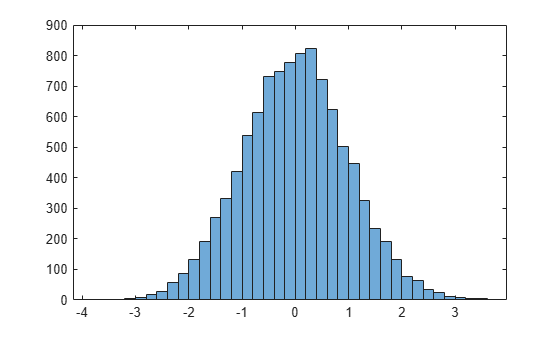```h = Histogram with properties: Data: [10000x1 double] Values: [1x37 double] NumBins: 37 BinEdges: [1x38 double] BinWidth: 0.2000 BinLimits: [-3.8000 3.6000] Normalization: 'count' FaceColor: 'auto' EdgeColor: [0 0 0] Show all properties ```

When you specify an output argument to the `histogram` function, it returns a histogram object. You can use this object to inspect the properties of the histogram, such as the number of bins or the width of the bins.

Find the number of histogram bins.

`nbins = h.NumBins`
```nbins = 37 ```

Plot a histogram of 1,000 random numbers sorted into 25 equally spaced bins.

```x = randn(1000,1); nbins = 25; h = histogram(x,nbins)```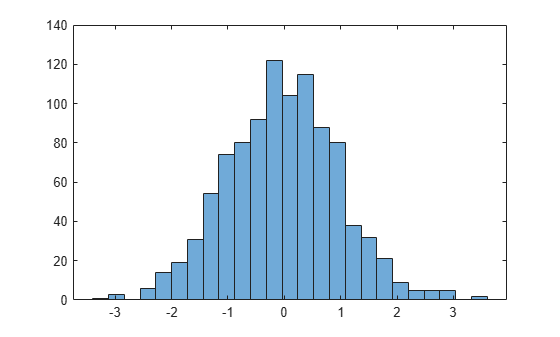```h = Histogram with properties: Data: [1000x1 double] Values: [1x25 double] NumBins: 25 BinEdges: [1x26 double] BinWidth: 0.2800 BinLimits: [-3.4000 3.6000] Normalization: 'count' FaceColor: 'auto' EdgeColor: [0 0 0] Show all properties ```

Find the bin counts.

`counts = h.Values`
```counts = 1×25 1 3 0 6 14 19 31 54 74 80 92 122 104 115 88 80 38 32 21 9 5 5 5 0 2 ```

Generate 1,000 random numbers and create a histogram.

```X = randn(1000,1); h = histogram(X)```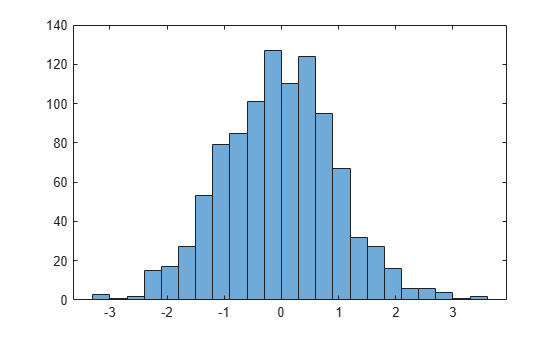```h = Histogram with properties: Data: [1000x1 double] Values: [1x23 double] NumBins: 23 BinEdges: [1x24 double] BinWidth: 0.3000 BinLimits: [-3.3000 3.6000] Normalization: 'count' FaceColor: 'auto' EdgeColor: [0 0 0] Show all properties ```

Use the `morebins` function to coarsely adjust the number of bins.

```Nbins = morebins(h); Nbins = morebins(h)```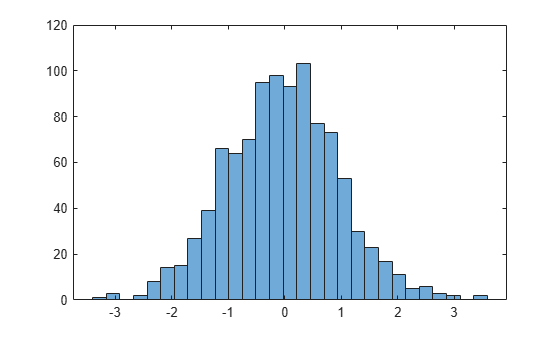```Nbins = 29 ```

Adjust the bins at a fine grain level by explicitly setting the number of bins.

`h.NumBins = 31;`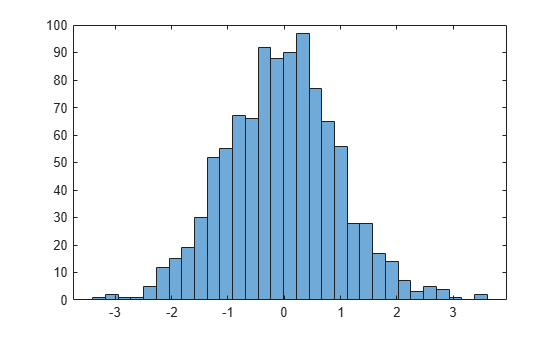Generate 1,000 random numbers and create a histogram. Specify the bin edges as a vector with wide bins on the edges of the histogram to capture the outliers that do not satisfy $|x|<2$. The first vector element is the left edge of the first bin, and the last vector element is the right edge of the last bin.

```x = randn(1000,1); edges = [-10 -2:0.25:2 10]; h = histogram(x,edges);```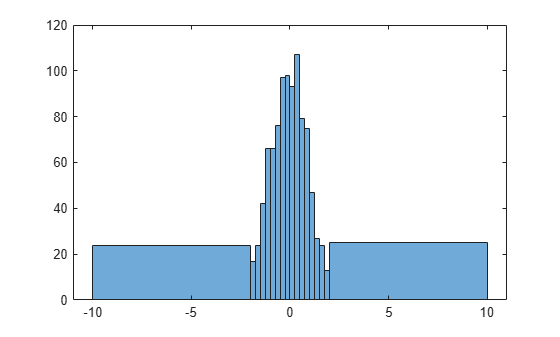Specify the `Normalization` property as `'countdensity'` to flatten out the bins containing the outliers. Now, the area of each bin (rather than the height) represents the frequency of observations in that interval.

`h.Normalization = 'countdensity';`Create a categorical vector that represents votes. The categories in the vector are `'yes'`, `'no'`, or `'undecided'`.

```A = [0 0 1 1 1 0 0 0 0 NaN NaN 1 0 0 0 1 0 1 0 1 0 0 0 1 1 1 1]; C = categorical(A,[1 0 NaN],{'yes','no','undecided'})```
```C = 1x27 categorical Columns 1 through 9 no no yes yes yes no no no no Columns 10 through 16 undecided undecided yes no no no yes Columns 17 through 25 no yes no yes no no no yes yes Columns 26 through 27 yes yes ```

Plot a categorical histogram of the votes, using a relative bar width of `0.5`.

`h = histogram(C,'BarWidth',0.5)`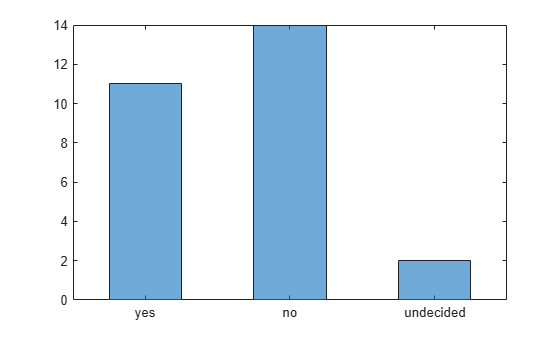```h = Histogram with properties: Data: [1x27 categorical] Values: [11 14 2] NumDisplayBins: 3 Categories: {'yes' 'no' 'undecided'} DisplayOrder: 'data' Normalization: 'count' DisplayStyle: 'bar' FaceColor: 'auto' EdgeColor: [0 0 0] Show all properties ```

Generate 1,000 random numbers and create a histogram using the `'probability'` normalization.

```x = randn(1000,1); h = histogram(x,'Normalization','probability')```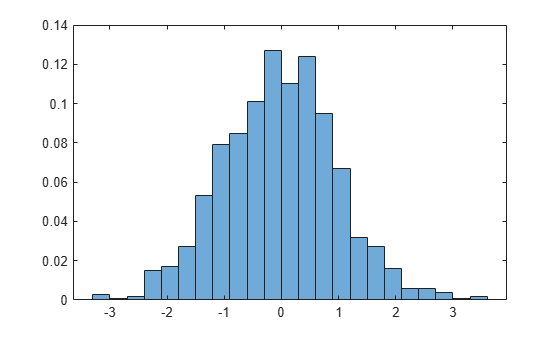```h = Histogram with properties: Data: [1000x1 double] Values: [1x23 double] NumBins: 23 BinEdges: [1x24 double] BinWidth: 0.3000 BinLimits: [-3.3000 3.6000] Normalization: 'probability' FaceColor: 'auto' EdgeColor: [0 0 0] Show all properties ```

Compute the sum of the bar heights. With this normalization, the height of each bar is equal to the probability of selecting an observation within that bin interval, and the height of all of the bars sums to 1.

`S = sum(h.Values)`
```S = 1 ```

Generate two vectors of random numbers and plot a histogram for each vector in the same figure.

```x = randn(2000,1); y = 1 + randn(5000,1); h1 = histogram(x); hold on h2 = histogram(y);```Since the sample size and bin width of the histograms are different, it is difficult to compare them. Normalize the histograms so that all of the bar heights add to 1, and use a uniform bin width.

```h1.Normalization = 'probability'; h1.BinWidth = 0.25; h2.Normalization = 'probability'; h2.BinWidth = 0.25;```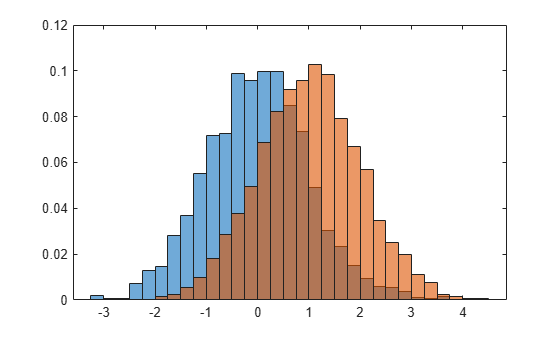Generate 1,000 random numbers and create a histogram. Return the histogram object to adjust the properties of the histogram without recreating the entire plot.

```x = randn(1000,1); h = histogram(x)```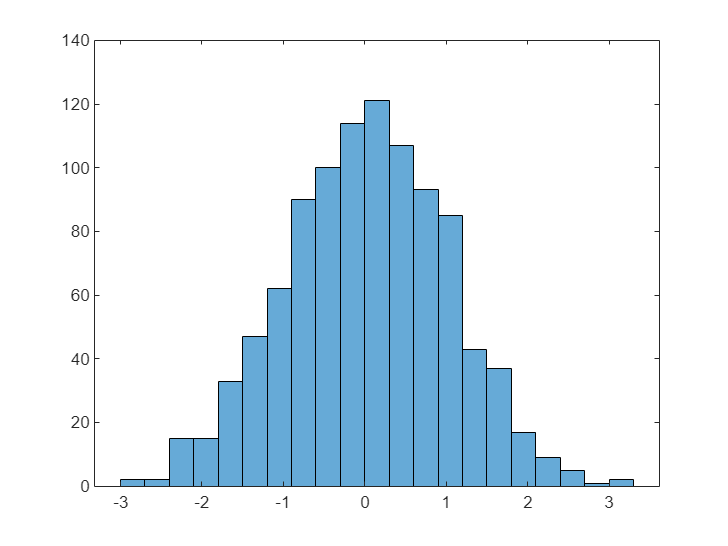```h = Histogram with properties: Data: [1000x1 double] Values: [1x23 double] NumBins: 23 BinEdges: [1x24 double] BinWidth: 0.3000 BinLimits: [-3.3000 3.6000] Normalization: 'count' FaceColor: 'auto' EdgeColor: [0 0 0] Show all properties ```

Specify exactly how many bins to use.

`h.NumBins = 15;`Specify the edges of the bins with a vector. The first value in the vector is the left edge of the first bin. The last value is the right edge of the last bin.

`h.BinEdges = [-3:3];`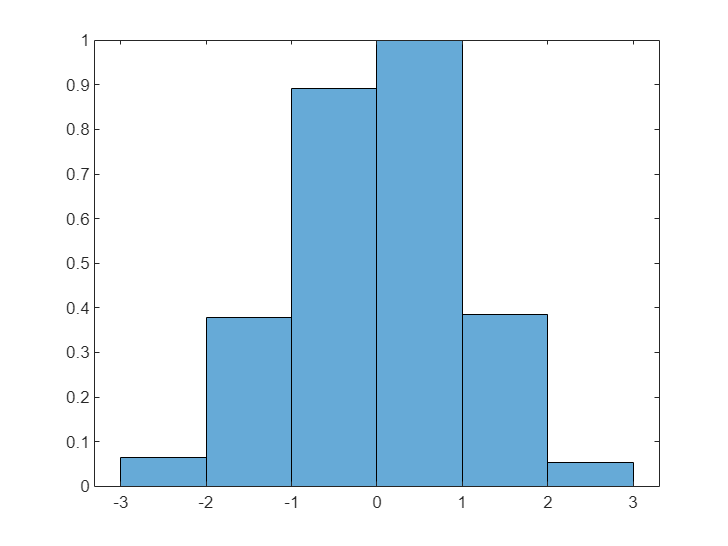Change the color of the histogram bars.

```h.FaceColor = [0 0.5 0.5]; h.EdgeColor = 'r';```Generate 5,000 normally distributed random numbers with a mean of 5 and a standard deviation of 2. Plot a histogram with `Normalization` set to `'pdf'` to produce an estimation of the probability density function.

```x = 2*randn(5000,1) + 5; histogram(x,'Normalization','pdf')```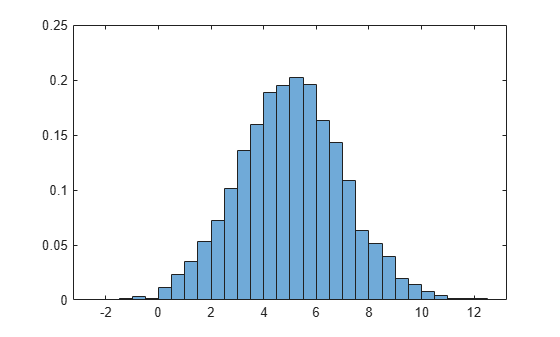In this example, the underlying distribution for the normally distributed data is known. You can, however, use the `'pdf'` histogram plot to determine the underlying probability distribution of the data by comparing it against a known probability density function.

The probability density function for a normal distribution with mean $\mu$, standard deviation $\sigma$, and variance ${\sigma }^{2}$ is

Overlay a plot of the probability density function for a normal distribution with a mean of 5 and a standard deviation of 2.

```hold on y = -5:0.1:15; mu = 5; sigma = 2; f = exp(-(y-mu).^2./(2*sigma^2))./(sigma*sqrt(2*pi)); plot(y,f,'LineWidth',1.5)```Use the `savefig` function to save a `histogram` figure.

```histogram(randn(10)); savefig('histogram.fig'); close gcf```

Use `openfig` to load the histogram figure back into MATLAB. `openfig` also returns a handle to the figure, `h`.

`h = openfig('histogram.fig');`Use the `findobj` function to locate the correct object handle from the figure handle. This allows you to continue manipulating the original histogram object used to generate the figure.

`y = findobj(h,'type','histogram')`
```y = Histogram with properties: Data: [10x10 double] Values: [2 17 28 32 16 3 2] NumBins: 7 BinEdges: [-3 -2 -1 0 1 2 3 4] BinWidth: 1 BinLimits: [-3 4] Normalization: 'count' FaceColor: 'auto' EdgeColor: [0 0 0] Show all properties ```

## Tips

• Histogram plots created using `histogram` have a context menu in plot edit mode that enables interactive manipulations in the figure window. For example, you can use the context menu to interactively change the number of bins, align multiple histograms, or change the display order.

• When you add data tips to a histogram plot, they display the bin edges and bin count.

## See Also

Introduced in R2014b

Download ebook